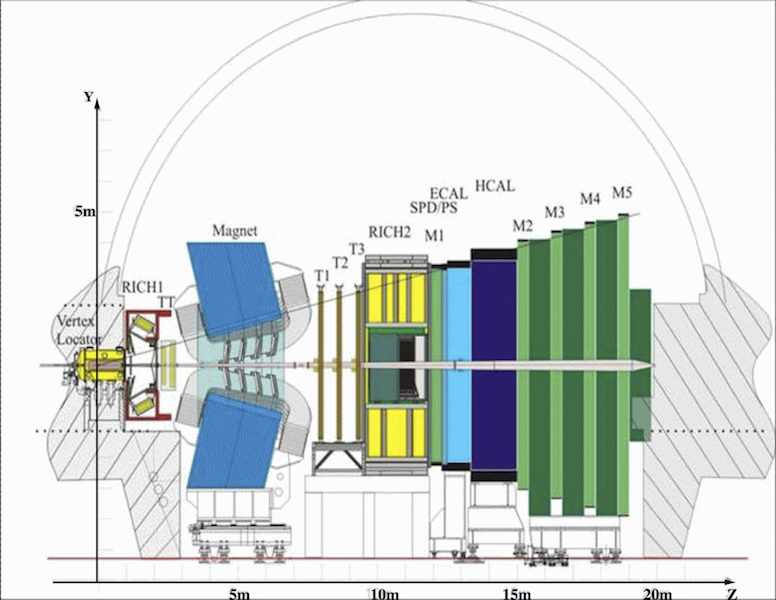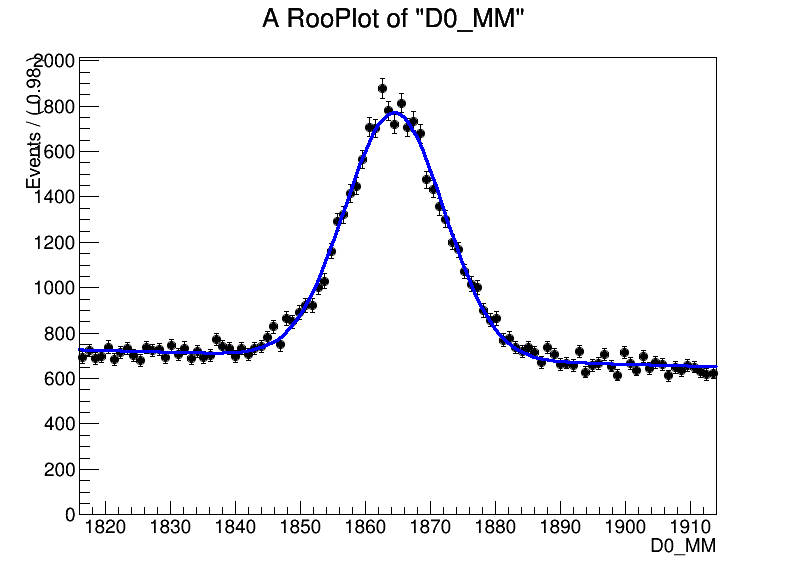# TESHEP practical work : Measuring the D0 lifetime at the LHC¶

## Introduction¶

The Large Hadron Collider (LHC) is not only a tool with which to search for exotic new particles, but also a factory of particles whose existence is in no doubt but whose precise properties are not yet known well enough. One example are charmed hadrons, i.e. hadrons which contain a c quark, which were first discovered more than 30 years ago. Approximately one in every ten LHC interactions produces a charmed hadron, and the LHCb experiment at the LHC has already recorded over one billion signal events in various decay channels. In order to extract information from such large signal samples, it is necessary to achieve excellent control over backgrounds. This set of exercises is designed to teach you how to...

The data sample used for this exercise consists of candidate $D^{0}$ mesons found in a sample of randomly collected LHC interactions during 2010 datataking. The mesons have been preselected using loose criteria so that you begin the exercise with a visible signal. A $D^{0}$ meson consists of a charm anti-quark and an up quark. The mesons are fully reconstructed decaying in the mode $D^{0}\to K^+ \pi^-$, where the final state particles are a kaon ($K^+$) consisting of a strange anti-quark and an up quark, and a pion ($\pi^-$) which consists of a down quark and an up anti-quark.Figure 1. The LHCb detector. The $\textbf{Z}$ axis is the direction of the LHC beamline.

Before discussing the data further, it is worth taking the time to familiarize yourselves with the LHCb detector, shown in Fig.1. It is a single-arm forward spectrometer covering the angular range between $0.7^\circ$ and $15^\circ$ relative to the beamline. In what follows transverse'' means transverse to the LHC beamline. The detector includes a high-precision tracking system consisting of a silicon-strip vertex detector surrounding the $pp$ interaction region, a large-area silicon-strip detector located upstream of a dipole magnet with a bending power of about $4{\rm\,Tm}$, and three stations of silicon-strip detectors and straw drift tubes placed downstream. The combined tracking system has a momentum resolution $\Delta p/p$ that varies from 0.4% at 5$~$GeV$/c$ to 0.6% at 100$~$GeV$/c$, an impact parameter resolution of 20 $\mu$m for tracks with high transverse momentum, and a decay time resolution of 50$~$fs. Charged hadrons are identified using two ring-imaging Cherenkov detectors. ...

## Exercise 1 : fitting the mass distribution¶

The object of this exercise is to fit the distribution of the $\textbf{D0_MM}$ variable and extract the signal yield and purity. If you plot this variable within the range (1816-1914)$~$MeV, you will see a peak (signal) on top of a flat distribution (background). The peak should be described by a Gaussian function, whose mean corresponds to the mass of the $D^0$ and whose width is determined by the experimental resolution of the LHCb detector. You should use the RooFit fitting framework for the following exercise.

Let's plot mass distribution of signal and background

In :
from ROOT import TFile, RooRealVar, RooDataSet, RooArgSet, RooGaussian, RooChebychev, RooArgList, RooAddPdf, RooFit
import rootnotes

In :
inputfile = TFile("TESHEP_Pedagogical_Data.root")

In :
inputchain = inputfile.Get("DecayTree")

In :
nameoffitvar = "D0_MM"

In :
rangeoffit = (1816., 1914)

In :
backgroundrange = (1890., 1914.)

In :
nsigmasigbox = 2.

In :
massvar = RooRealVar("D0_MM", "D0_MM", rangeoffit, rangeoffit)

In :
mydataset = RooDataSet("DecayTreeDataSet","DecayTreeDataSet",RooArgSet(massvar))

In :
for entry in range(0,inputchain.GetEntries()) :
inputchain.GetEntry(entry)
if inputchain.__getattr__(nameoffitvar) > rangeoffit or \
inputchain.__getattr__(nameoffitvar) < rangeoffit :
continue
massvar.setVal(inputchain.__getattr__(nameoffitvar))

In :
gausMean_B 	= RooRealVar("gausMean_B","gausMean_B",(rangeoffit+rangeoffit)/2.,rangeoffit,rangeoffit)
gausWidth_B 	= RooRealVar("gausWidth_B","gausWidth_B",15.,1.,50.)
chebOne_B 	= RooRealVar("chebOne_B","chebOne_B",0.1,-5.,5.)
gaussian_B 	= RooGaussian("gaussian_B","gaussian_B",massvar,gausMean_B,gausWidth_B)
cheb_B     	= RooChebychev("cheb_B","cheb_B",massvar,RooArgList(chebOne_B))

In :
N_Sig_B = RooRealVar("N_Sig_B","N_Sig_B",10000,0,250000)
N_Bkg_B = RooRealVar("N_Bkg_B","N_Bkg_B",10000,0,500000)

In :
totalpdf_B = RooAddPdf("totalpdf_B","totalpdf_B", RooArgList(gaussian_B,cheb_B), RooArgList(N_Sig_B,N_Bkg_B))
totalpdf_B.fitTo(mydataset, RooFit.Extended())

Out:
<ROOT.RooFitResult object at 0x(nil)>
In :
canv_Dmass = rootnotes.default_canvas()
frame_Dmass = massvar.frame()
mydataset.plotOn(frame_Dmass,RooFit.Binning(100))
totalpdf_B.plotOn(frame_Dmass)
frame_Dmass.Draw()

In :
canv_Dmass

Out:In :
massvar.setRange("integralRangeAll", rangeoffit, rangeoffit)
massvar.setRange("integralRangeSignal",
gausMean_B.getVal() - nsigmasigbox * gausWidth_B.getVal(),
gausMean_B.getVal() + nsigmasigbox * gausWidth_B.getVal())
massvar.setRange("integralRangeBackground", backgroundrange, backgroundrange)

In :
integralall    = cheb_B.createIntegral(RooArgSet(massvar), RooFit.NormSet(RooArgSet(massvar)), RooFit.Range("integralRangeAll"))
integralsignal = cheb_B.createIntegral(RooArgSet(massvar), RooFit.NormSet(RooArgSet(massvar)), RooFit.Range("integralRangeSignal"))
integralback   = cheb_B.createIntegral(RooArgSet(massvar), RooFit.NormSet(RooArgSet(massvar)), RooFit.Range("integralRangeBackground"))

In :
normalizedIntegralValue_S = integralsignal.getVal()/integralall.getVal()
normalizedIntegralValue_B = integralback.getVal()/integralall.getVal()

In :
print "Signal range =",\
gausMean_B.getVal() - nsigmasigbox * gausWidth_B.getVal(), "-",\
gausMean_B.getVal() + nsigmasigbox*gausWidth_B.getVal()
print "Background in signal range =", normalizedIntegralValue_S * N_Bkg_B.getVal()
print "Background in background range =",normalizedIntegralValue_B * N_Bkg_B.getVal()

print "Background scale factor =", normalizedIntegralValue_S / normalizedIntegralValue_B

Signal range = 1849.15867581 - 1879.81505391
Background in signal range = 21579.3283653
Background in background range = 16197.0885418
Background scale factor = 1.33229674639

In [ ]: